• ## 偏微分

千次阅读 2019-09-11 09:52:44
最近开始看人工智能，发现自己的数学知识已经忘的差不多了，为了自己不至于越学习越迷茫，于是自己简单整理了下，也算对这几天...对偏微分符号不熟悉(多看看概念就懂了，回想下大学是学过的，只不过忘了) 下面是...
最近开始看人工智能，发现自己的数学知识已经忘的差不多了，为了自己不至于越学习越迷茫，于是自己简单整理了下，也算对这几天努力学习的一个总结。 先说下之前我的一些认识误区:
认为微积分是类似积分的一种数学方法。(实际上是微分和积分的合称)对dx和Δx傻傻分不清区别(dx是切线上y轴的增量，而Δx是y函数上微小的增量)对偏微分符号不熟悉(多看看概念就懂了，回想下大学是学过的，只不过忘了)
下面是自己摘录的概念和总结
一阶导数即切线的斜率，通常理解即x上的一个微小增量和对应y轴上微小增量的比值。斜率并非一个角度的概念，在正方向上，越接近y轴，越趋向无穷大，核心理解在于微小增量上。微分是指函数图像在某一点处的切线在横坐标取得增量Δx以后，纵坐标取得的增量，一般表示为dy，这里注意是切线。偏微分是指一个方程中有多个变量，如果我们对其中一个变量求导，假设其他变量不变的话。这样即针对这一个变量的偏导，即偏微分。范数即向量的长度，是一个标量。用||两根竖线来表示。
这些数学知识的学习中，我一开始陷入了想大而全的迷茫中，而忽视了一个一个知识点去突破，最终这样效果很差。 另外善于总结非常重要，不然会忘记的很快，精读模式，一定要理解透彻，再进入下一个知识点的学习中。
展开全文• 古典显式格式求解抛物型偏微分方程（一维热传导方程）
• 偏微分方程丘成桐偏微分方程丘成桐偏微分方程丘成桐偏微分方程丘成桐
• 偏微分方程：指含有多元未知函数u=u(x),x=(x1,x2,...,Xn)u=u(x),x=(x_1,x_2,...,X_n)u=u(x),x=(x1​,x2​,...,Xn​)及其若干阶偏导数的关系式 F(x,u,∂u∂x1,∂u∂x2,...,∂u∂xn,...,∂mu∂x1m1∂x2m2...∂xnmn)=0 ...
偏微分方程：指含有多元未知函数

u

=

u

(

x

)

,

x

=

(

x

1

,

x

2

,

.

.

.

,

X

n

)

u=u(x),x=(x_1,x_2,...,X_n)

及其若干阶偏导数的关系式

F

(

x

,

u

,

∂

u

∂

x

1

,

∂

u

∂

x

2

,

.

.

.

,

∂

u

∂

x

n

,

.

.

.

,

∂

m

u

∂

x

1

m

1

∂

x

2

m

2

.

.

.

∂

x

n

m

n

)

=

0

F(\bold x,u,\frac{\partial u}{\partial x_1},\frac{\partial u}{\partial x_2},...,\frac{\partial u}{\partial x_n},...,\frac{\partial^m u}{\partial x_1^{m_1}\partial x_2^{m_2}...\partial x_n^{m_n}})=0

其中，最高阶导数的阶数

m

=

m

1

+

m

1

+

.

.

.

+

m

n

m=m_1+m_1+...+m_n

为方程的阶。
线性偏微分方程：偏微分方程中与未知函数有关的部分是

u

u

及

u

u

的偏导数的线性组合（系数与

u

u

或

u

u

的偏导数无关）。
常系数线性微分方程：方程中u和u的偏导数的系数是常数
意义：偏微分方程反映了变量u及多个自变量

x

=

(

x

1

,

x

2

,

.

.

.

,

x

n

)

\bold x=(x_1,x_2,...,x_n)

间的相互制约关系。
数学物理方程：从物理问题中导出的偏微分方程称为数学物理中的偏微分方程。有时还包括常微分方程和积分方程。
偏微分方程的定解问题：泛定方程+定解条件
泛定方程
波动方程 ：

∂

2

u

∂

t

2

=

a

2

Δ

u

+

f

(

t

,

x

→

)

,

a

=

T

ρ

,

f

(

t

,

x

→

)

=

g

(

t

,

x

→

)

ρ

\frac{\partial^2 u}{\partial t^2}=a^2\Delta u+f(t,\overrightarrow x),a=\sqrt{\frac{T}{\rho}},f(t,\overrightarrow x)=\frac{g(t,\overrightarrow x)}{\rho}

扩散方程：

∂

u

∂

t

=

a

2

Δ

u

+

f

(

t

,

x

→

)

,

a

=

κ

c

ρ

,

f

(

t

,

x

→

)

=

g

(

t

,

x

→

)

c

ρ

\frac{\partial u}{\partial t}=a^2\Delta u+f(t,\overrightarrow x),\space a=\sqrt{\frac{\kappa}{c\rho}},f(t,\overrightarrow x)=\frac{g(t,\overrightarrow x)}{c\rho}

场位方程：

Δ

u

=

−

f

(

x

)

x

=

(

x

1

,

x

2

,

.

.

.

,

x

n

)

,

n

=

1

,

2

,

3

定解条件
初始条件（历史情况的影响）  边界条件（周围环境对边界的影响） 第I类边界条件（给顶端点值）：

u

∣

x

=

x

i

=

μ

i

(

t

)

u|_{x=x_i}=\mu_i(t)

第II类边界条件（给定端点梯度）：

∂

u

∂

n

∣

x

=

x

i

=

f

i

(

t

)

\frac{\partial u}{\partial n}|_{x=x_i}=f_i(t)

第III类边界条件（混合I&II）：

[

a

i

u

+

β

i

∂

u

∂

n

]

x

=

x

i

=

F

i

(

t

)

[a_iu+\beta_i\frac{\partial u}{\partial n}]_{x=x_i}=F_i(t)

衔接条件（系统内部边界）
实际应用：找出泛定方程+定解条件，然后利用多种方法解偏微分方程
展开全文• 偏微分方程ppt讲义，结合数理方法，建立偏微分方程
• 俄罗斯数学教材选译-偏微分方程讲义(第3版)+偏微分方程习题集.rar
• 使用偏微分方程进行图像去噪,图像处理的偏微分方程方法,matlab源码
• 偏微分方程的数值解法的程序,偏微分方程数值解法答案,matlab源码
• 偏微分题目，程序作业。。。。。。。。。。。。。。。。。。。。。。
• 偏微分方程复习整理 偏微分方程中的基本知识 经典偏微分方程 定解问题与定解条件 等等有关PDE的知识点归纳
• 偏微分方程的数值解法,偏微分方程的数值解法有哪些,C,C++源码
• Matlab偏微分方程快速上手：使用pdetool工具箱求解二维偏微分方程，适用于数学建模、数学实验，简单的偏微分方程数值计算与工程问题。
注：本人使用MatlabR2020a版本。
1.pdetoolbox的调用
打开MatlabR2020a，在命令行键入pdetool，进入pdetoolbox。2.绘制定解区域（解的定义域）
由图形界面可知，解的定义域是

x

,

y

x,y

二维坐标构成的平面空间。我们必须设置自己的定解区域，才能定义自己的方程： 导航栏下方的前5个按钮，分别对应绘制矩形求解区域、绘制按中心生成的矩形求解区域、绘制椭圆形(圆形)定解区域、绘制按中心生成的椭圆形(圆形)定解区域、绘制多边形求解区域。使用时，只需要点击后在绘图区域拖拽(多边形除外，多边形区域是在绘图区域点点以确定顶点)，就可以生成定解区域了。上面这是前五个按钮。 在这里，作者随意绘制了一个椭圆形区域，和一个矩形区域。操作的时候用鼠标拖动操作柄拖拽就可以。（也可以画好几个叠起来）真的是“随便”画一个就可以哦，因为在matlab下，求解区域的位置坐标精度达到了

1

0

−

16

10^{-16}

左右，手动画几乎不可能画准。所以下一步教大家怎么细致地调节边界的坐标。
3.手动调整定解区域的大小
双击刚刚绘制好的区域，弹出一个对话框，里面是我们的定解区域的边界坐标信息（注意不全是坐标），我们可以在这里手动调整定解区域的位置（以矩形区域为例）：这就是刚刚打开时的样子。因为这个区域是作者随便画的，所以坐标信息就像随机数一样。下面我们输入精确的数值：Left: -1, Bottom: -1, Width: 2, Height: 2, Name 就用默认的就好。参数的意义：Left：左边界的坐标(x左)，Bottom：底边界的坐标(y底)，Width：区域宽度，Height：区域高度。这样就得到了

x

∈

[

−

1

,

1

]

,

y

∈

[

−

1

,

1

]

x\in[-1,1],y\in[-1,1]

的矩形求解区域。调整好的求解区域显示效果如下。4.调整绘图窗口的显示区域（调整显示坐标限）
有时候我们会发现我们的定解区域太大了，绘图窗口显示不下；或者定解区域太小了，看上去非常不协调。上一个例子中作者的纵坐标显然非常吻合，但是横坐标多出来了（显示了左右两边的白框），那么我强烈建议大家调整完定解区域的坐标以后，再调整一下绘图窗口的显示区域。 点击导航栏Options，再点击Axes Limits…(意为调整坐标限)，可以手动设置坐标限。这里作者勾选了Auto，这样matlab将自动帮我们调整坐标限，使得定解区域位于界面中央。Options还有其他操作，大家可以自己尝试一下，这里就不介绍了。调整后的效果如下。5.确定边界条件
点击导航栏下方第6个按钮(

∂

Ω

\partial \Omega

，意为

Ω

\Omega

的边界条件)，它用来显示边界。下面仍然以矩形区域为例。此时显示了4个边界。双击任意一个边界，会弹出边界条件对话框，可以在这里随意设置边界条件。在这里可以设置Dirichlet边界条件、Neumann边界条件和Robin边界条件（分别是第一、第二、第三边界条件），其中Robin边界条件和Neumann边界条件集成到一起了。按提示输入对应的系数就可。
在这里作者使用了如下的边界条件：

x

=

±

1

,

u

=

0

;

y

=

±

1

,

∂

u

∂

n

=

0.

x=\pm1,u=0;y=\pm1,\frac{\partial u}{\partial n }=0.

如果用了Dirichlet边界条件，边界将显示为红色；Neumann、Robin边界条件将显示蓝色，效果如下。6.确定偏微分方程的形式
点击第7个图标（显示PDE字样），按提示输入偏微分方程的系数即可。在这里笔者求解波动方程：

∂

2

u

∂

2

t

=

∇

u

.

\frac{\partial^2 u}{\partial^2 t}=\nabla u.工具箱提供的方程通式如下： 1.椭圆型Elliptic，通用数学形式为

−

∇

⋅

(

c

∇

u

)

+

a

u

=

f

;

-\nabla \cdot(c\nabla u)+au=f ;

2.抛物型Parabolic，通用数学形式为

d

∂

u

∂

t

−

∇

⋅

(

c

∇

u

)

+

a

u

=

f

;

d\frac{\partial u}{\partial t}-\nabla \cdot(c\nabla u)+au=f ;

3.双曲型Hyperbolic，通用数学形式为

d

∂

2

u

∂

2

t

−

∇

⋅

(

c

∇

u

)

+

a

u

=

f

;

d\frac{\partial^2 u}{\partial^2 t}-\nabla \cdot(c\nabla u)+au=f ;

4.特征值方程Eigenmodes，若

λ

\lambda

为特征值，则数学形式为

−

∇

⋅

(

c

∇

u

)

+

a

u

=

λ

d

u

.

-\nabla \cdot(c\nabla u)+au=\lambda d u .

也可以自行指定求解的方程类型，比如比较常见的热传导、扩散等方程，可以在下面图示的下拉菜单中选择，但是仍然要按上面讲的方法手动设置系数。7.三角剖分
由于Matlab pdetoolbox使用有限元方法求解，所以需要三角剖分。点击第8个图标（1个三角形图样）可以初始化剖分，点击第9个图标（4个三角形图样）可以增加剖分密度，这样可以提高计算精度，但是密度过高内存可能会爆掉，使用要谨慎。8.设置初始条件，准备求解
点击导航栏Solve，再点击Parameters…，进入求解参数设置器。在这里，第一行Time我们可以设置

t

t

的求解范围及步长，默认情况下是不显示步长的（默认显示0:10意为从0求解到10，步长为1），我们按照Maltab等差数列的生成方法 a:j:b 就可以设置时间步长j了。
第二行u(t0)、第三行u’(t0)表示

t

0

t_0

时刻的两个初始条件。这里作者使用了如下的初始条件。第四行和第五行表示相对容差和绝对容差，笔者查看了Matlab帮助中心，大概了解到这两个参数似乎与浮点数0的截断精度有关，太小的话会延长计算时间，如果你想了解更多，笔者把链接提供上来Absolute tolerance - MATLAB & Simulink - MathWorks 中国，假如我们对计算精度没有要求的话，使用默认值就可以了。这里笔者为了演示使用了0.001和0.0001。如果想跟着一起做，那么笔者把方程的代码也放上来：第一个是atan(cos(pi/2*x))，第二个是3*sin(pi*x).*exp(cos(pi*y))。
9.求解
点击导航栏下方按钮(一个“

=

=

”字样的按钮，就是增加三角剖分密度右边那个按钮)，这个按钮表示开始求解。如果求解完成的话会显示这个图。在这里可以点击“放大镜”按钮寻找感兴趣的区域放大来观察细节（放大之后想要缩小就要用上面步骤4的方法重新设置坐标限了，没有找到缩小的快捷键）。它直接显示了

t

=

10

t=10

时

u

u

在

求

解

区

域

Ω

求解区域\Omega

的图像。这样的输出缺乏直观性，我们点击导航栏下方一个长得像matlab的logo的按钮（就是“

=

=

”按钮右边那个），调整绘图格式。这个窗口有许多功能，作者就不再一一详述了。大家可以自行调试。比较常用的有“Contour”绘制等高线图，“Arrows”绘制向量场，“Height(3-D plot)”按3D模式输出（这个比较常用），“Animation”按动画形式输出(2D\3D都支持)，此选项勾选后右边的Option选项会变亮，我们可以点进去在里面设置1秒显示的帧数、重复播放的次数。这里作者按照25阶变色、‘jet’ Colormap、向量场为

−

∇

u

-\nabla u

的形式静态输出

t

=

0.5

t=0.5

时的结果，如下图所示。这就是matlab pdetool工具箱的主要使用方法，本人也是小白一枚，所以欢迎大家批评指正，可以在评论区留下你的想法。
参考：偏微分方程（姜礼尚《数学物理方程讲义》第三版）（更新完毕，附课件）来自于西北大学数统学院的马老师的数理方程视频课，是按数学系的讲法讲的数理方程，里面有那么两三个视频是讲如何用Matlab求解偏微分方程，如果你懂偏微分方程的话进去听一遍就会了。 感觉应该是疫情期间这位老师的网课视频？那么西北大学的学生们也太幸福了，因为马老师讲的真的很好！人也很好玩哈哈，还去b站里别的老师的微分几何课程下面评论，正巧那个被评论的老师的同学就在b站讲拓扑哈哈，那个老师讲的也特别细我还给听完了(浙江理工庄老师)。扯远了！但是还是安利！！！
展开全文matlab 数学建模
• 偏微分方程的数值解系列博文： 偏微分方程的数值解(一):定解问题 & 差分解法 偏微分方程的数值解(二): 一维状态空间的偏微分方程的 MATLAB 解法 偏微分方程的数值解(三): 化工应用实例 ----------触煤反应...
偏微分方程的数值解系列博文：
偏微分方程的数值解(一):定解问题 & 差分解法
偏微分方程的数值解(二): 一维状态空间的偏微分方程的 MATLAB 解法
偏微分方程的数值解(三): 化工应用实例 ----------触煤反应装置内温度及转换率的分布
偏微分方程的数值解(四): 化工应用————扩散系统之浓度分布
偏微分方程的数值解(五)： 二维状态空间的偏微分方程的 MATLAB 解法
偏微分方程的数值解(六)： 偏微分方程的 pdetool 解法

目录
1 图形界面解法简介
2 图形界面解法的使用步骤

1 图形界面解法简介
对于一般的区域，任意边界条件的偏微分方程，我们可以利用 MATLAB 中 pdetool 提供的偏微分方程用户图形界面解法。 图形界面解法步骤大致上为：
（1）定义 PDE 问题，包括二维空间范围，边界条件以及 PDE 系数等。
（2）产生离散化之点，并将原 PDE 方程式离散化。
（3）利用有限元素法(finite element method；FEM)求解并显示答案。
在说明此解法工具之前，先介绍此 PDE 图形界面的菜单下方的功能图标(icon)按 钮。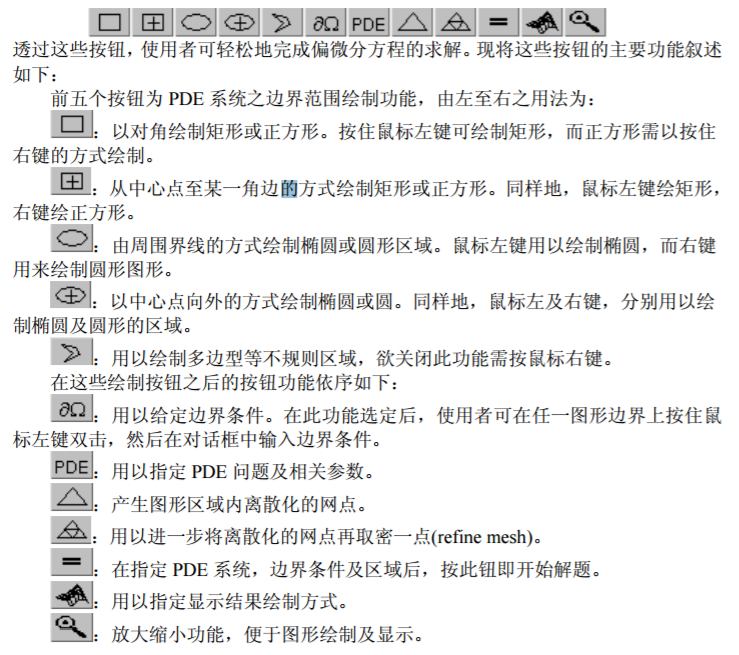2 图形界面解法的使用步骤
要利用 pdetool 接口求解之前，需先定义 PDE 问题，其包含三大部份：
（1）利用绘图(draw)模式，定义 需要求解的问题的空间范围(domain)Ω 。
（2）利用 boundary 模式，指定边界条件。
（3）利用 PDE 模式，指定 PDE 系数，即输入 c，a，f 和 d 等 PDE 模式中的系数。
在定义 PDE 问题之后，可依以下两个步骤求解
（1）在 mesh 模式下，产生 mesh 点，以便将原问题离散化。
（2）在 solve 模式下，求解。
（3）最后，在 Plot 模式下，显示答案。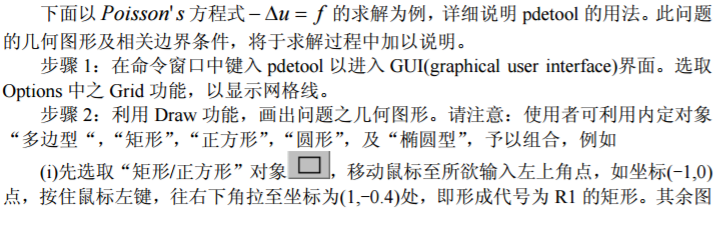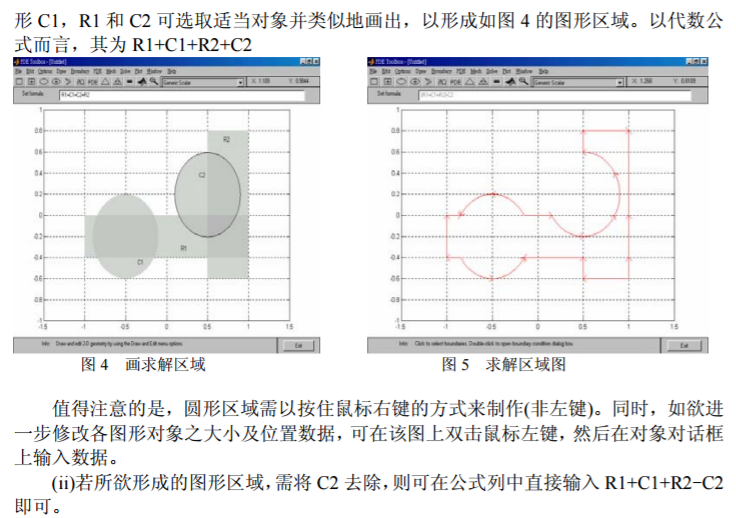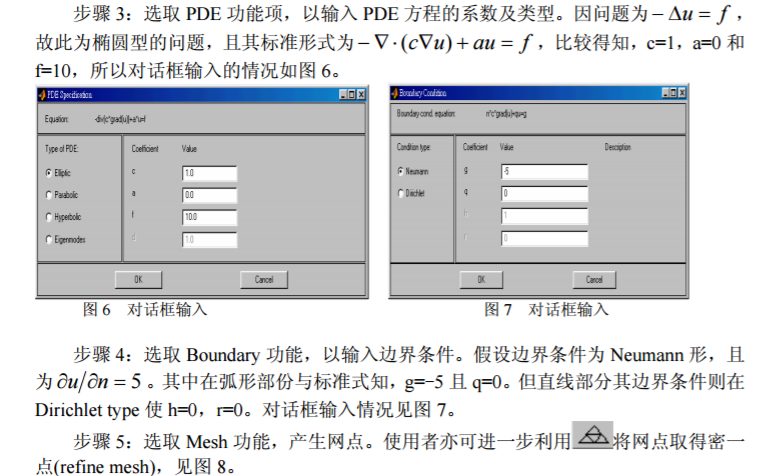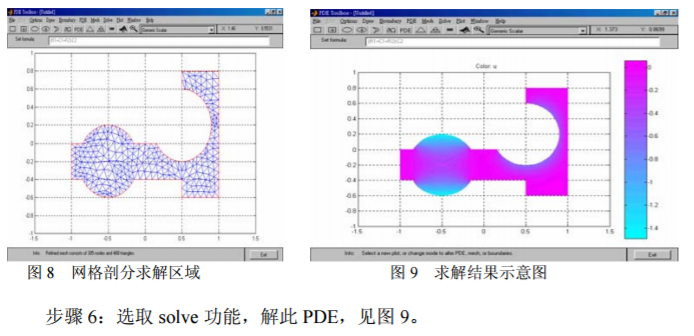注意：
1. MATLAB 会以图形的方式展示结果，使用者亦可点选 plot 下之“parameters”功 能，选择适当的方式显示图形及数据。例如用 3D 方式显示求解结果。参数设置见图 10， 显示结果见图 11。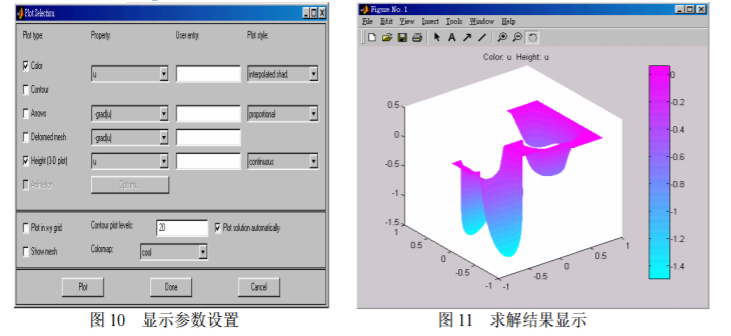2. 另外，若使用者欲将结果输出到命令窗口中，以供后续处理，可利用 solve 功能 项下之“export solution”指定变量名称来完成。
3. 如果求抛物型或双曲型方程的数值解，还需要通过“solve”菜单下的 “parameters…”选项设置初值条件。
4. 在上面定义边界条件和初始条件时，可以使用一些内置变量。
（1）在边界条件输入框中，可以使用如下变量： 二维坐标 x 和 y，边界线段长度参数（s s 是以箭头的方向沿边界线段从 0 增加到 1）， 外法向矢量的分量 nx 和 ny（如果需要边界的切线方向，可以通过 tx=-ny 和 ty=nx 表示）， 解 u。
（2）在初值条件的输入框中，也可以输入用户定义的 MATLAB 可接受变量（p， e，t，x，y）的函数。

例 11 使用 PDETOOL 重新求例 8 的数值解。
例8  求解正方形区域{(x, y) | −1 ≤ x, y ≤ 1}上的热传导方程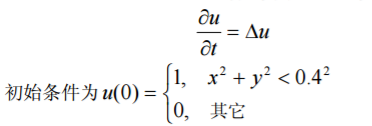边界条件为Dirichlet条件u = 0。
解 这里是抛物型方程，其中c = 1, a = 0, f = 0, d = 1。
1）定义 PDE 问题，包括二维空间范围，边界条件以及 PDE 系数等。我们这里就 省略了。
2）区域剖分以后，通过“Mesh”菜单下的“Export Mesh…”选项可以把 p，e，t 三个参数分别输出到工作间。
3）然后编写函数 fun1(x,y)如下：
function f=fun1(x,y);
f=zeros(length(x),1);
ix=find(x.^2+y.^2<0.16);
f(ix)=1; 
其中的变量 x，y 是 MATLAB 可接受的内置变量。 设置“solve”菜单下的“parameters…”选项如下：
时间框中输入：linspace(0,0.1,20)；
初值框中输入：fun1。
4）设置“plot"菜单下的“parameters…”选项如下：选择 Height(3-D plot) 和 Animation 两项。
5）用鼠标点一下工具栏上的“＝”按钮，就可以画出数值解的 3-D 图形。


展开全文• Matlab偏微分方程的数值解法常用程序-偏微分方程的数值解法_程序.rar 包括解决一些解偏微分方程的常用程序，希望对大家有用，欢迎下载！！:)matlab
• 偏微分方程的几种数值解法M程序-偏微分方程的数值解法.rar 未命名.JPGmatlab
• 偏微分方程绪论部分，包括二阶片偏微分方程的分类，解的特性等
• 结合MATLAB偏微分方程数值解工具箱介绍偏微分方程的求解，分GUI和MATLAB函数两种实现方式进行介绍。matlab Matlab 编程语言
• 基于椭圆型偏微分方程生成二维NACA0012翼型的O型网格并求解流场
• 分享偏微分方程的MATLAB解法pdf-偏微分方程的MATLAB解法.part1.rar 处理偏微分方程时可以参考参考，希望对大家有帮助^_^目录： Figure40.jpg Figure41.jpg ...matlab
• 分享偏微分方程的MATLAB解法pdf-偏微分方程的MATLAB解法.part3.rar 处理偏微分方程时可以参考参考，希望对大家有帮助^_^目录： Figure40.jpg Figure41.jpg ...matlab
• 分享偏微分方程的MATLAB解法pdf-偏微分方程的MATLAB解法.part2.rar 处理偏微分方程时可以参考参考，希望对大家有帮助^_^目录： Figure40.jpg Figure41.jpg ...matlab
• 偏微分习题讲解，非常不错的书，适合初学者，经常练习...